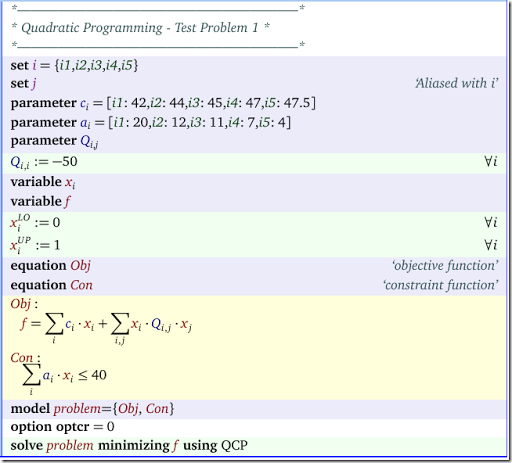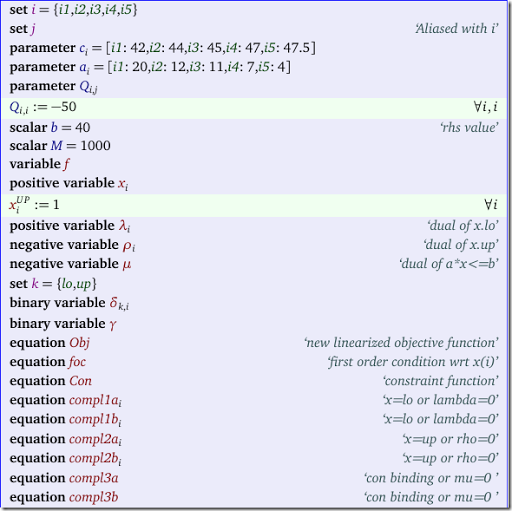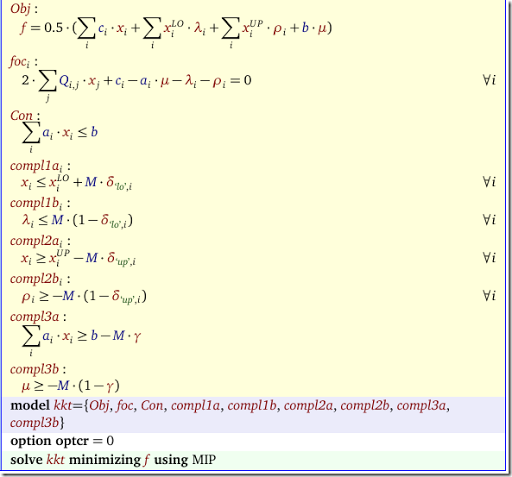## Saturday, June 18, 2016

### Solving non-convex QP problems as a MIP

Non-convex Quadratic Programming problems are difficult to solve to optimality. Most QP solvers do not even accept non-convex problems, so how can we attack this?

#### The easy way: global solvers

The easiest is just to use a global solver. We can use global NLP solvers such as Baron, Qouenne or Antigone. In addition Cplex has a solver for non-convex QP and MIQP problems (you need to set an option for this: OptimalityTarget = 3; Cplex will not automatically use this non-convex solver). For these solvers make sure to set the gap tolerance (GAMS option OPTCR) to zero.

#### Theory: KKT Conditions

A QP model can look like:

 \begin{align}\min\>\> & c^Tx + x^TQx \\&Ax \le b\\& \ell \le x \le u \end{align}

The KKT conditions for this QP are:

 \begin{align} & 2Qx + c - A^T\mu – \lambda - \rho = 0\\&(Ax – b)^T\mu = 0\\&(x-\ell)^T\lambda = 0 \\&(x-u)^T\rho = 0\\& \ell \le x \le u \\ & Ax \le b \\& \lambda \ge 0,\rho \le 0, \mu \le 0 \end{align}

There are two complications with this LCP (Linear Complementarity Problem):

• The complementarity conditions $$(Ax – b)^T\mu$$, $$(x-\ell)^T\lambda=0$$ and  $$(x-u)^T\rho$$ are not linear and can not be used directly in an LP or MIP model
• There are likely multiple solutions, so we need a way to select the best

#### A MIP formulation

To make the above model amenable to a linear solver we first try to linearize the complementarity conditions. Let’s consider $$(x-\ell)^T\lambda=0$$. This can be attacked as follows. The condition $$(x_i –\ell_i)\lambda_i = 0$$ really means $$x_i=\ell_i$$ or $$\lambda_j=0$$. This can be linearized as:

 \begin{align} & x_i \le \ell_i +M \delta_i\\& \lambda_i \le M (1-\delta_i) \\& \delta_i \in \{0,1\} \end{align}

We can do something similar for $$(x-u)^T\rho$$ and $$(Ax – b)^T\mu$$.

The big-M coefficient are a problem of course. We either need to find some good values for them or use a formulation that does not need them. Some techniques that could help here are SOS1 (Special Order Sets of Type 1) variables where each combination $$y_i, \lambda_i$$ (where $$y_i=x_i-\ell_i$$ forms a SOS1 set. An alternative is to use some indicator variables (these are supported by a number of solvers).

The second issue is to find the best solution. Obviously we could add an objective $$\min\> c^Tx + x^TQx$$ but that makes the problem non-linear again. It turns out that $$c^Tx + x^TQx = \frac{1}{2} \left[ c^Tx +\ell^T\lambda + u^T\rho+ b^T\mu\right]$$. This is now a linear objective!

#### Objective

The derivation of the linear objective is as follows:

 \begin{align} & c^Tx + x^TQx\\= \>& c^Tx + \frac{1}{2} x^T ( \lambda + \rho + A^T\mu – c)\\= \> & c^Tx + \frac{1}{2}x^T\lambda + \frac{1}{2}x^T\rho + \frac{1}{2}(Ax)^T\mu – \frac{1}{2}x^Tc\\=\> & \frac{1}{2} c^Tx + \frac{1}{2}\ell^T\lambda + \frac{1}{2}u^T\rho + \frac{1}{2} b^T\mu \end{align}

It is quite fortunate that we are able to get rid of the quadratic term and form a linear objective.

#### Example: Non-convex QP

A simple example is from (1):Solving this directly gives:

 LOWER          LEVEL          UPPER         MARGINAL ---- EQU Obj                 .              .              .             1.0000      ---- EQU Con               -INF           39.0000        40.0000          .            Obj  objective function  Con  constraint function ---- VAR x            LOWER          LEVEL          UPPER         MARGINAL i1          .             1.0000         1.0000       -58.0000      i2          .             1.0000         1.0000       -56.0000      i3          .              .             1.0000        45.0000      i4          .             1.0000         1.0000       -53.0000      i5          .              .             1.0000        47.5000                                 LOWER          LEVEL          UPPER         MARGINAL ---- VAR f                 -INF          -17.0000        +INF             .

#### Example: MIP with big-M coefficients

We name our duals as follows:

 \begin{align} x_i \ge \ell_i & \perp \lambda_i \ge 0 \\ x_i \le u_i & \perp \rho_i \le 0 \\ \sum_i a_i x_i \le b & \perp \mu \le 0\end{align}

The complete model looks like:The results look like:

 LOWER          LEVEL          UPPER         ---- VAR f                 -INF          -17.0000        +INF                      ---- VAR x            LOWER          LEVEL          UPPER         i1          .             1.0000         1.0000             i2          .             1.0000         1.0000             i3          .              .             1.0000             i4          .             1.0000         1.0000             i5          .              .             1.0000             ---- VAR lambda  dual of x.lo           LOWER          LEVEL          UPPER         i1          .              .            +INF                       i2          .              .            +INF                       i3          .            45.0000        +INF                       i4          .              .            +INF                       i5          .            47.5000        +INF                       ---- VAR rho  dual of x.up           LOWER          LEVEL          UPPER         i1        -INF          -58.0000          .                        i2        -INF          -56.0000          .                        i3        -INF             .              .                        i4        -INF          -53.0000          .                        i5        -INF             .              .                                                   LOWER          LEVEL          UPPER         ---- VAR mu                -INF             .              .                         mu  dual of a*x<=b ---- VAR delta               LOWER          LEVEL          UPPER         lo.i1          .             1.0000         1.0000             lo.i2          .             1.0000         1.0000             lo.i3          .              .             1.0000                lo.i4          .             1.0000         1.0000             lo.i5          .              .             1.0000                up.i1          .              .             1.0000                up.i2          .              .             1.0000                up.i3          .             1.0000         1.0000             up.i4          .              .             1.0000                up.i5          .             1.0000         1.0000                                        LOWER          LEVEL          UPPER         ---- VAR gamma               .             1.0000         1.0000

For some performance comparisons see (3).

#### References

(1)  Floudas e.a., Handbook of Test Problems in Local and Global Optimization, Kluwer, 1999.
(2)  Wei Xia, Juan Vera, Louis F. Zuluaga, Globally Solving Non-Convex Quadratic Programs via Linear Integer Programming Techniques, report, Nov 17, 2015.
(3)  http://yetanothermathprogrammingconsultant.blogspot.com/2016/07/non-convex-qp-as-mip-performance.html

#### 1 comment:

1.